# Chapter 6 Arrays 1 Fall 2012 CS 2302

• Slides: 16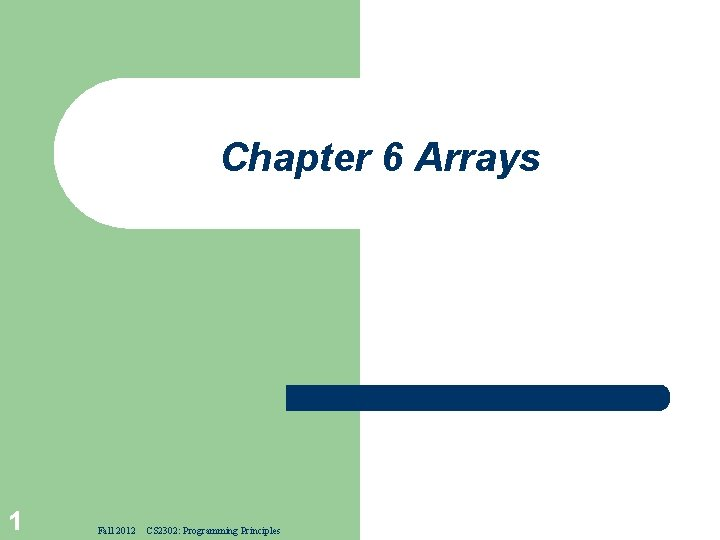Chapter 6 Arrays 1 Fall 2012 CS 2302: Programming PrinciplesWhy array? § Store a large number of values during the execution of a program. § What is array? – 2 Fall 2012 A fixed-size sequential collection of elements of the same type. CS 2302: Programming Principles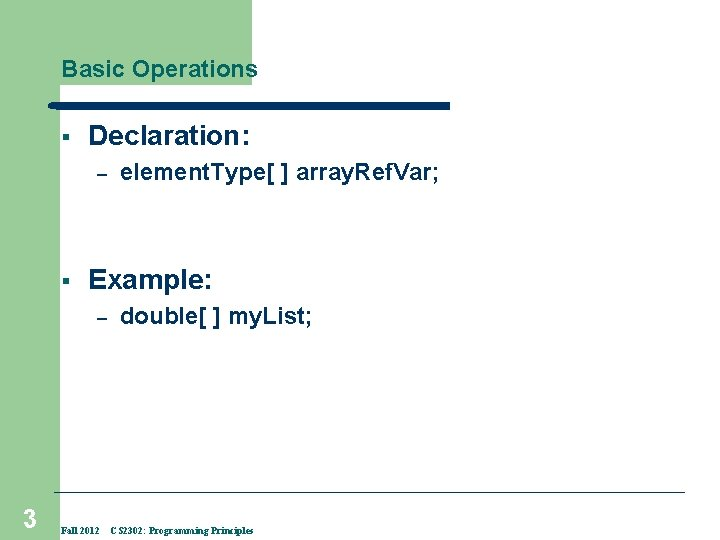Basic Operations § Declaration: – § Example: – 3 element. Type[ ] array. Ref. Var; Fall 2012 double[ ] my. List; CS 2302: Programming Principles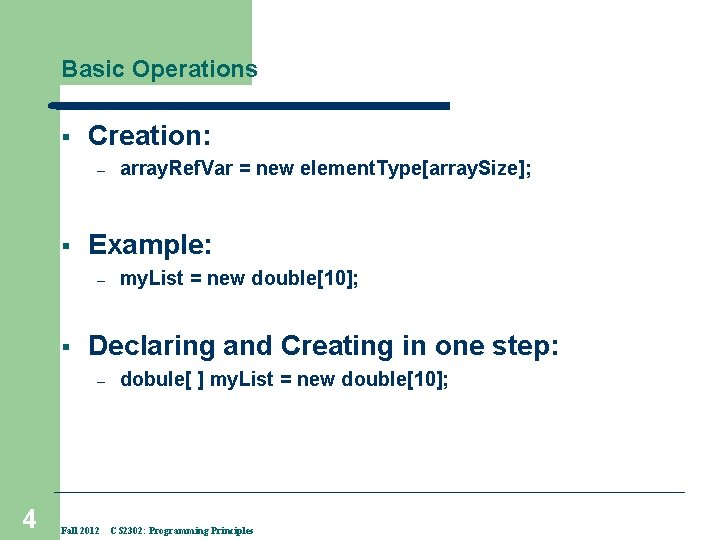Basic Operations § Creation: – § Example: – § my. List = new double; Declaring and Creating in one step: – 4 array. Ref. Var = new element. Type[array. Size]; Fall 2012 dobule[ ] my. List = new double; CS 2302: Programming Principles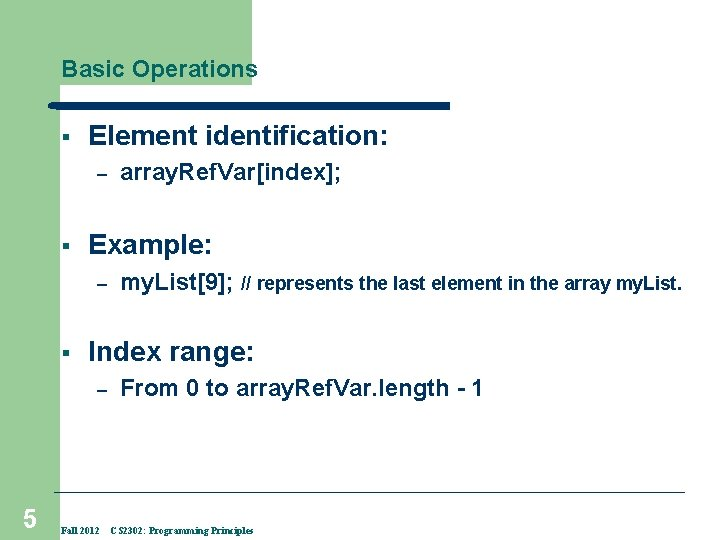Basic Operations § Element identification: – § Example: – § my. List; // represents the last element in the array my. List. Index range: – 5 array. Ref. Var[index]; Fall 2012 From 0 to array. Ref. Var. length - 1 CS 2302: Programming Principles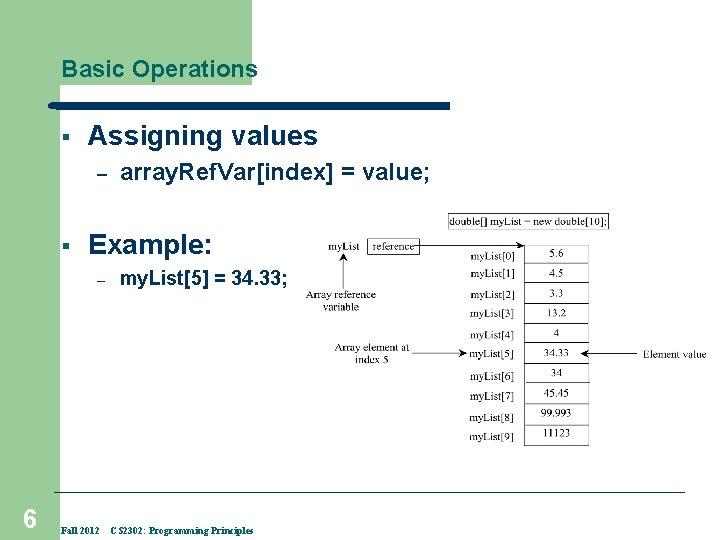Basic Operations § Assigning values – § Example: – 6 array. Ref. Var[index] = value; Fall 2012 my. List = 34. 33; CS 2302: Programming Principles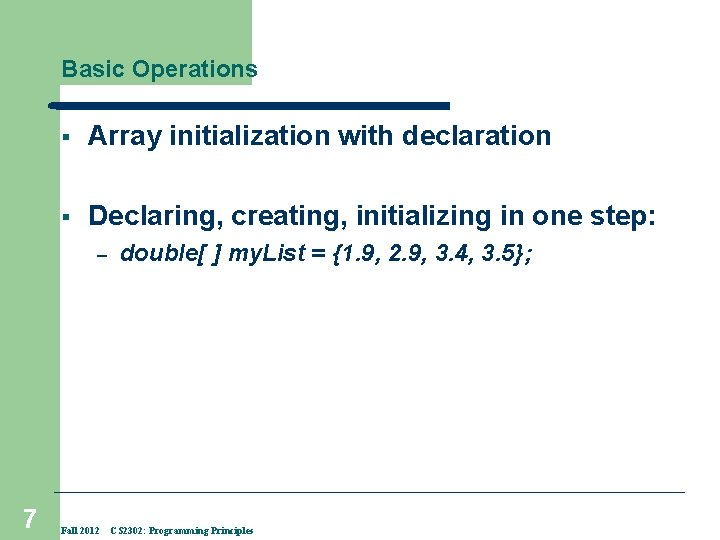Basic Operations § Array initialization with declaration § Declaring, creating, initializing in one step: – 7 Fall 2012 double[ ] my. List = {1. 9, 2. 9, 3. 4, 3. 5}; CS 2302: Programming Principles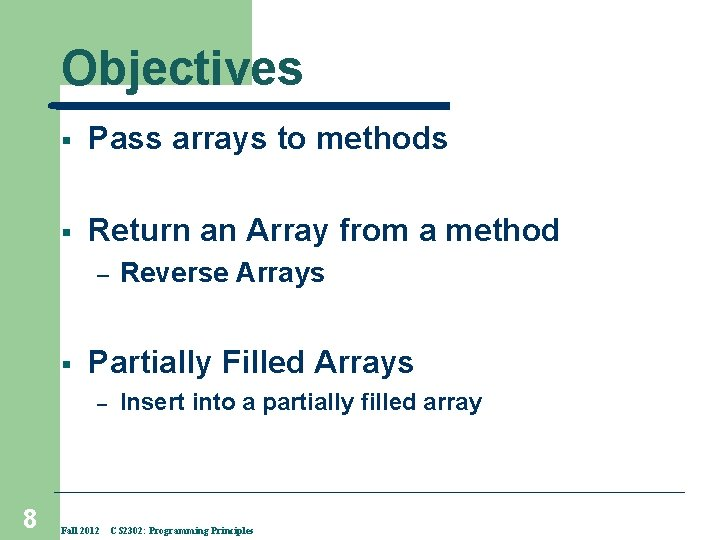Objectives § Pass arrays to methods § Return an Array from a method – § Partially Filled Arrays – 8 Reverse Arrays Fall 2012 Insert into a partially filled array CS 2302: Programming PrinciplesPass by value Most methods are passed arguments when they are called. An argument may be a constant or a variable. § Math. sqrt(33) § § 9 Math. sqrt(x) If a variable is passed, the method receives a copy of the variable's value. The value of the original variable cannot be changed within the method. § because the method only has a copy of the value; it does not have access to the original variable. This process is called pass by value. Fall 2012 CS 2302: Programming PrinciplesPass by Value 10 public class Test. Pass. By. Value { /** Main method */ public static void main(String[] args) { // Declare and initialize variables int num 1 = 1; int num 2 = 2; System. out. println("Before invoking the swap method, num 1 is " + num 1 + " and num 2 is " + num 2); // Invoke the swap method to attempt to swap two variables swap(num 1, num 2); System. out. println("After invoking the swap method, num 1 is " + num 1 + " and num 2 is " + num 2); } /** swap method - Swap two variables */ public static void swap(int n 1, int n 2) { System. out. println("t. Inside the swap method"); System. out. println("tt. Before swapping n 1 is " + n 1 + " n 2 is " + n 2); // Swap n 1 with n 2 int temp = n 1; n 1 = n 2 = temp; System. out. println("tt. After swapping n 1 is " + n 1 + " n 2 is " + n 2); } } Fall 2012 CS 2302: Programming PrinciplesPassing Arrays to Methods public static void print. Array(int[] array) { for (int i = 0; i < array. length; i++) { System. out. print(array[i] + " "); } } //Invoke the method int[] list = {3, 1, 2, 6, 4, 2}; //initialize array print. Array(list); //Invoke the method print. Array(new int[]{3, 1, 2, 6, 4, 2}); Anonymous array 11 Fall 2012 CS 2302: Programming Principles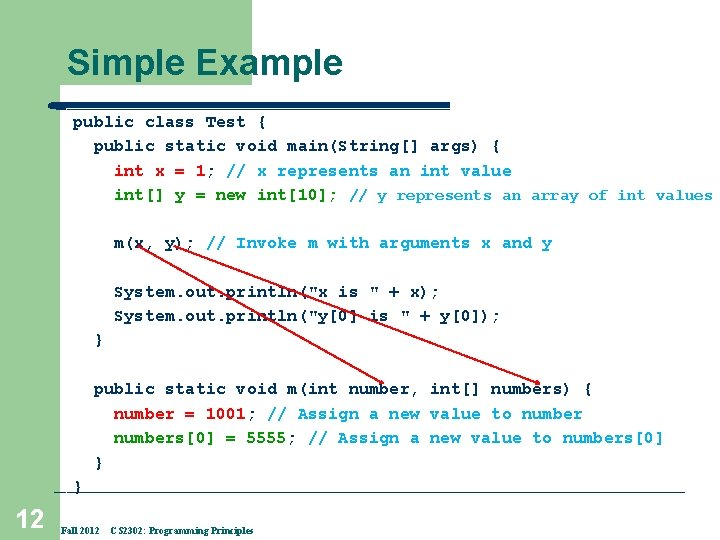Simple Example public class Test { public static void main(String[] args) { int x = 1; // x represents an int value int[] y = new int; // y represents an array of int values m(x, y); // Invoke m with arguments x and y System. out. println("x is " + x); System. out. println("y is " + y); } public static void m(int number, int[] numbers) { number = 1001; // Assign a new value to numbers = 5555; // Assign a new value to numbers } } 12 Fall 2012 CS 2302: Programming Principles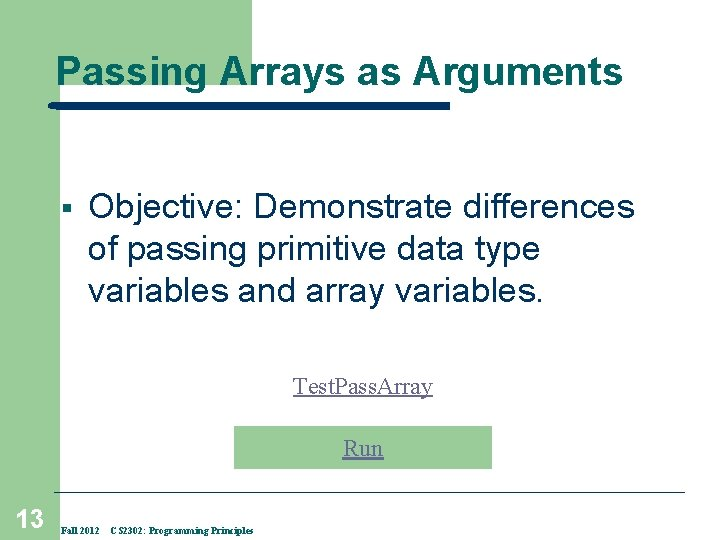Passing Arrays as Arguments § Objective: Demonstrate differences of passing primitive data type variables and array variables. Test. Pass. Array Run 13 Fall 2012 CS 2302: Programming Principles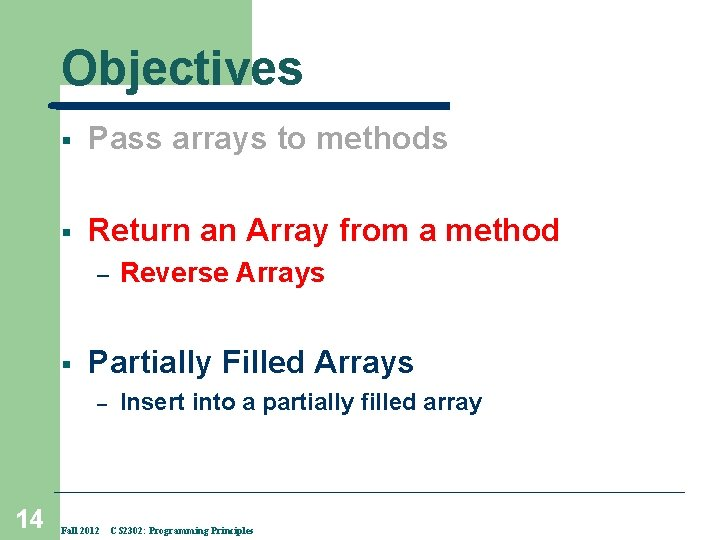Objectives § Pass arrays to methods § Return an Array from a method – § Partially Filled Arrays – 14 Reverse Arrays Fall 2012 Insert into a partially filled array CS 2302: Programming Principles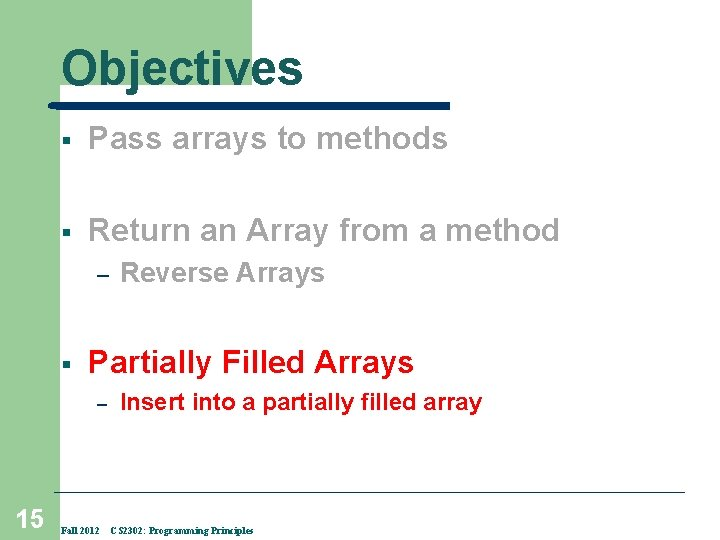Objectives § Pass arrays to methods § Return an Array from a method – § Partially Filled Arrays – 15 Reverse Arrays Fall 2012 Insert into a partially filled array CS 2302: Programming Principles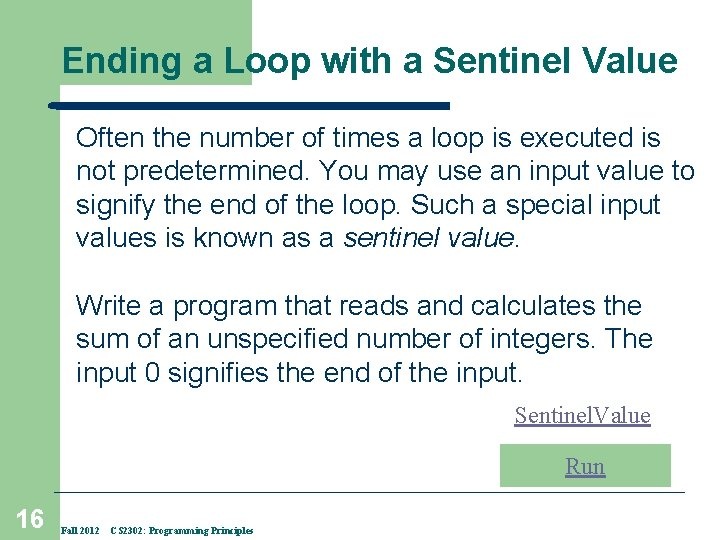Ending a Loop with a Sentinel Value Often the number of times a loop is executed is not predetermined. You may use an input value to signify the end of the loop. Such a special input values is known as a sentinel value. Write a program that reads and calculates the sum of an unspecified number of integers. The input 0 signifies the end of the input. Sentinel. Value Run 16 Fall 2012 CS 2302: Programming Principles